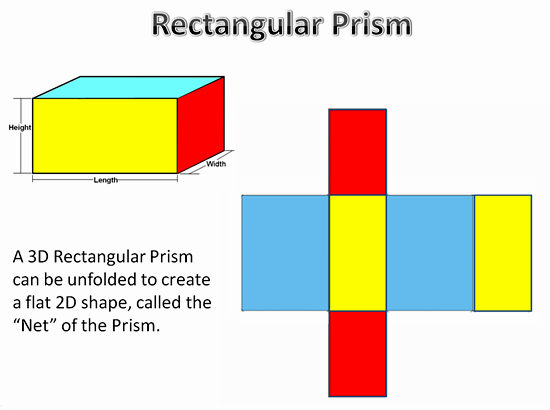# What is the surface area formula of a 3-dimensional rectangle?

Jan 9, 2016

$\text{SA} = 2 \left(w l + l h + h w\right)$

#### Explanation:

For a rectangular prism with sides $w , l , h$, the surface area is

$\text{SA} = 2 \left(w l + l h + h w\right)$This occurs since there are two pairs of three different faces on every rectangular prism.

Each pair of faces is a different rectangle using two of the the three dimensions of the prism as its own side.

One side is just $w l$, another is just $l h$, and the other $h w$. Since there are two of each, that is reflected in the formula by the multiplication by $2$.

This could also be imagined as a series of flattened-out rectangles:The blue rectangles are $2 \cdot w l$.

The yellow rectangles are $2 \cdot l h$.

The red rectangles are $2 \cdot h w$.

Again, the surface area would be

$\text{SA} = 2 w l + 2 l h + 2 h w$

$= 2 \left(w l + l h + h w\right)$